Nuclear Physics Nat Level – 1

# Nuclear Physics Nat Level – 1 - Physics

Test Description

## 10 Questions MCQ Test Topic wise Tests for IIT JAM Physics - Nuclear Physics Nat Level – 1

Nuclear Physics Nat Level – 1 for Physics 2023 is part of Topic wise Tests for IIT JAM Physics preparation. The Nuclear Physics Nat Level – 1 questions and answers have been prepared according to the Physics exam syllabus.The Nuclear Physics Nat Level – 1 MCQs are made for Physics 2023 Exam. Find important definitions, questions, notes, meanings, examples, exercises, MCQs and online tests for Nuclear Physics Nat Level – 1 below.
Solutions of Nuclear Physics Nat Level – 1 questions in English are available as part of our Topic wise Tests for IIT JAM Physics for Physics & Nuclear Physics Nat Level – 1 solutions in Hindi for Topic wise Tests for IIT JAM Physics course. Download more important topics, notes, lectures and mock test series for Physics Exam by signing up for free. Attempt Nuclear Physics Nat Level – 1 | 10 questions in 30 minutes | Mock test for Physics preparation | Free important questions MCQ to study Topic wise Tests for IIT JAM Physics for Physics Exam | Download free PDF with solutions
 1 Crore+ students have signed up on EduRev. Have you?
*Answer can only contain numeric values
Nuclear Physics Nat Level – 1 - Question 1

### The half life of a radioactive substance is 3h and its activity is 1µCi. Then the activity after 9h will be (in µCi)

Detailed Solution for Nuclear Physics Nat Level – 1 - Question 1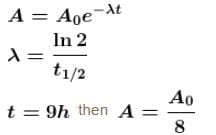*Answer can only contain numeric values
Nuclear Physics Nat Level – 1 - Question 2

### An electron positron pair (rest mass of each particle = 0.51 MeV) is produced by a γ -ray photon of energy 2.42 MeV. The K.E. (in MeV) imparted to each of the charged particles shall be :

Detailed Solution for Nuclear Physics Nat Level – 1 - Question 2

Rest mass energy + kinetic energy = energy of gamma photon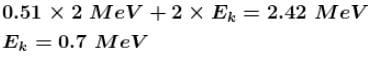*Answer can only contain numeric values
Nuclear Physics Nat Level – 1 - Question 3

### The probability of a radioactive atoms to survive 5 times longer than its half value period is.

Detailed Solution for Nuclear Physics Nat Level – 1 - Question 3

Decay period = Half life × No. of half lives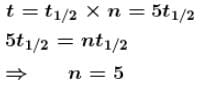*Answer can only contain numeric values
Nuclear Physics Nat Level – 1 - Question 4

A radioactive nuclie decay as follows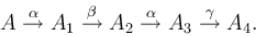If mass number and atomic number of A are 180 and 72 respectively then atomic number for A4 will be.

Detailed Solution for Nuclear Physics Nat Level – 1 - Question 4

72A180 -α->70A1176 -β->71A2176 -α->69A3172-γ->69A4174

*Answer can only contain numeric values
Nuclear Physics Nat Level – 1 - Question 5

After emission of an α -particle by a radioactive element 84X212, the resulting mass number would be.

Detailed Solution for Nuclear Physics Nat Level – 1 - Question 5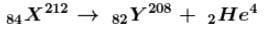*Answer can only contain numeric values
Nuclear Physics Nat Level – 1 - Question 6

If the mass of proton = 1.008 amu and mass of neutron = 1.009 amu then binding energy (in MeV) per nucleon for 4Be9  (mass = 9.012 amu) would be :

Detailed Solution for Nuclear Physics Nat Level – 1 - Question 6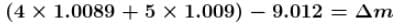Binding energy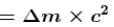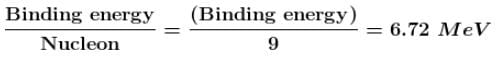*Answer can only contain numeric values
Nuclear Physics Nat Level – 1 - Question 7

After a time equal to four half lives, the percentage of radioactive material remaining undecayed is :

Detailed Solution for Nuclear Physics Nat Level – 1 - Question 7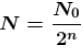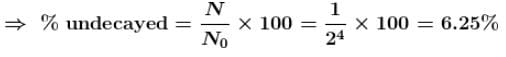*Answer can only contain numeric values
Nuclear Physics Nat Level – 1 - Question 8

The half-life of cobalt 60 is 5.25 years. How long after a new sample is delivered, will the activity have decreased to about one third (1/3) of its original value. (Provide the time in years).

Detailed Solution for Nuclear Physics Nat Level – 1 - Question 8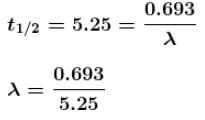*Answer can only contain numeric values
Nuclear Physics Nat Level – 1 - Question 9

The mean life of a radioactive sample is 100 years. Then after 100 years, what percentage of the sample remains active.

Detailed Solution for Nuclear Physics Nat Level – 1 - Question 9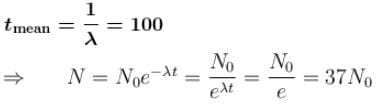*Answer can only contain numeric values
Nuclear Physics Nat Level – 1 - Question 10

1014 fissions per second are taking place in a nuclear reactor having efficiency 40%. The energy released per fission is 250 MeV. The power output (in Watts) of the reactor is :

Detailed Solution for Nuclear Physics Nat Level – 1 - Question 10

Power output 0.4 x power input = 0.4 x 250 x 106 x 1.6 x 10-19 x 1014 = 1600W.(Scan QR code)